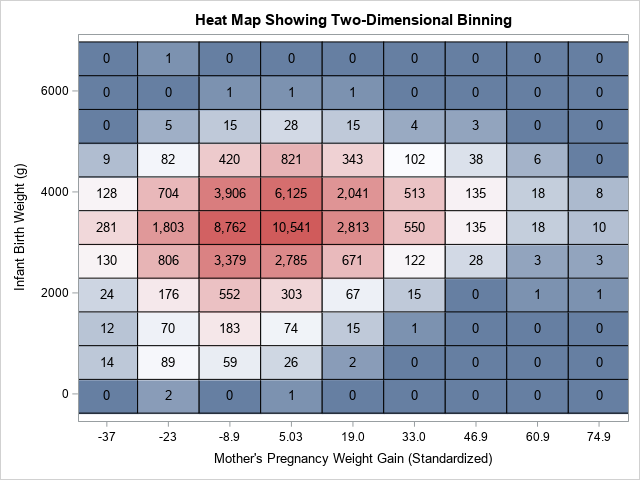Do you want to bin a numeric variable into a small number of discrete groups? This article compiles a dozen resources and examples related to binning a continuous variable. The examples show both equal-width binning and quantile binning. In addition to standard one-dimensional techniques, this article also discusses various techniques for 2-D binning.

SAS procedures that support binning include the HPBIN, IML, KDE, RANK, and UNIVARIATE procedures.

### Equal-width binning in SAS

The simplest binning technique is to form equal-width bins, which is also known as bucket binning. If a variable has the range [Min, Max] and you want to split the data into k equal-width bins (or buckets), each bin will have width (Max - Min) / k.

### Quantile binning in SAS

In bucket binning, some bins have more observations than others. This enables you to estimate the density of the data, as in a histogram. However, you might want all bins to contain about the same number of observations. In that case, you can use quantiles of the data as cutpoints. If you want four bins, use the 25th, 50th, and 75th percentiles as cutpoints. If you want 10 bins, use the sample deciles as cutpoints. Here are several resources for quantile binning:

### Binning by using arbitrary cutpoints in SAS

Sometimes you need to bin based on scientific standards or business rules. For example, the Saffir-Simpson hurricane scale uses specific wind speeds to classify a hurricane as Category 1, Category 2, and so forth. In these cases, you need to be able to define custom cutpoints and assign observations to bins based on those cutpoints.

### 2-D binning and bivariate histograms in SAS

A histogram is a visualization of a univariate equal-width binning scheme. You can perform similar computations and visualizations for two-dimensional data. If your goal is to understand the density of continuous bivariate data, you might want to use a bivariate histogram rather than a scatter plot (which, for large samples, suffers from overplotting).

In summary, this guide provides many links to programs and examples that bin data in SAS. Whether you want to use equal-width bins, quantile bins, or two-dimensional bins, hopefully, you will find an example to get you started. If I've missed an important topic, or if you have a favorite binning method that I have not covered, leave a comment.

The post The essential guide to binning in SAS appeared first on The DO Loop.